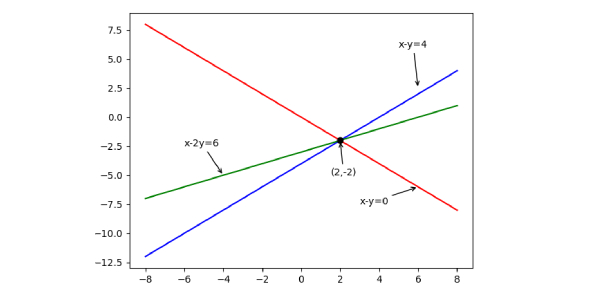Linear Equation Exam Quiz: Trivia!

13 Questions | Total Attempts: 370Settings.

• 1.
Given the graph of a linear equation above, write the equation for the line in General form (y=a+bx).
• 2.
Given the slope and y intercept above, write the equation for the line in General form (y=a+bx).
• 3.
Given the slope and y intercept above, write the equation for the line in General form (y=a+bx).
• 4.
Given the slope and y intercept above, write the equation for the line in General form (y=a+bx).
• 5.
Given the slope and y intercept above, write the equation for the line in General form (y=a+bx).
• 6.
Given the slope and y intercept above, write the equation for the line in General form (y=a+bx).
• 7.
Given the slope and y intercept above, write the equation for the line in General form (y=a+bx).
• 8.
Given the slope and y intercept above, write the equation for the line in General form (y=a+bx).
• 9.
Given the slope and y intercept above, write the equation for the line in General form (y=a+bx).
• 10.
Given the slope and y intercept above, write the equation for the line in General form (y=a+bx).
• 11.
Given the slope and y intercept above, write the equation for the line in General form (y=a+bx).
• 12.
Given the graph of a linear equation above, write the equation for the line in General form (y=a+bx).
• 13.
Given the graph of a linear equation above, write the equation for the line in General form (y=a+bx).
• 14.
Given the graph of a linear equation above, write the equation for the line in General form (y=a+bx).
• 15.
Given the graph of a linear equation above, write the equation for the line in General form (y=a+bx).
• 16.
Given the graph of a linear equation above, write the equation for the line in General form (y=a+bx).
• 17.
Given the graph of a linear equation above, write the equation for the line in General form (y=a+bx).
• 18.
Given the graph of a linear equation above, write the equation for the line in General form (y=a+bx).
• 19.
Given the graph of a linear equation above, write the equation for the line in General form (y=a+bx).
• 20.
Given the graph of a linear equation above, write the equation for the line in General form (y=a+bx).
• 21.
Given the graph of a linear equation above, write the equation for the line in General form (y=a+bx).
• 22.
Gradient = 2,    Point on the line: (1, 4)Given the gradient/slope and a point on the line above, write the equation for the line in General form (y=a+bx).
• 23.
Gradient = 5,    Point on the line: (1, 4)Given the gradient/slope and a point on the line above, write the equation for the line in General form (y=a+bx).
• 24.
Gradient = 2,    Point on the line: (1, 3)Given the gradient/slope and a point on the line above, write the equation for the line in General form (y=a+bx).
• 25.
Gradient = -3,    Point on the line: (1, 3)Given the gradient/slope and a point on the line above, write the equation for the line in General form (y=a+bx).
Related TopicsBack to top